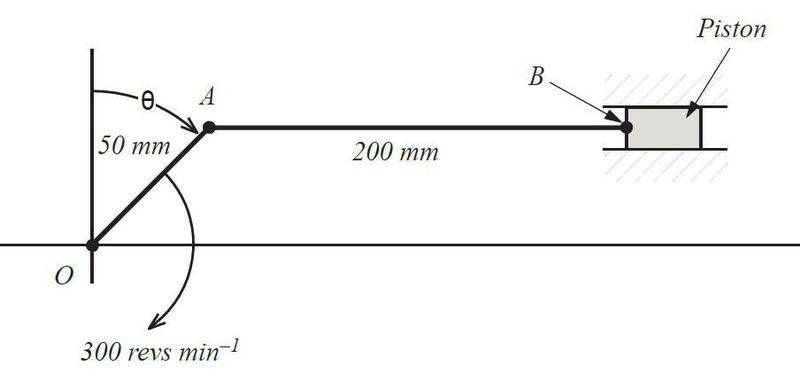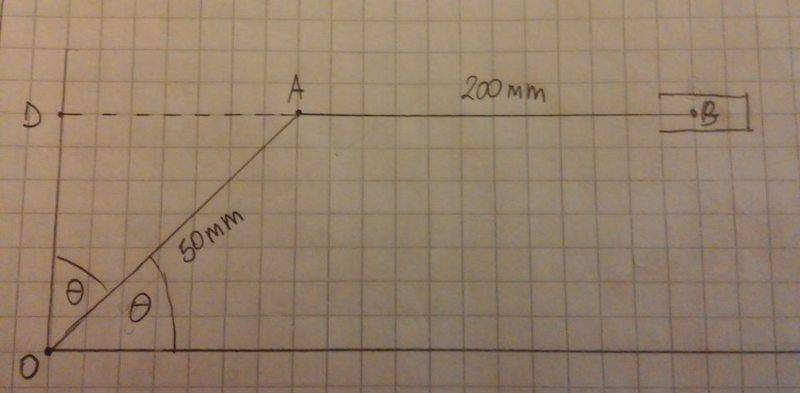# Offset crank slider mechanism connecting rod analysis

## Homework Statement

A) Determine the value of the angle θ (measured from vertical) when the angular velocity of link AB a maximum.

B) What is the maximum angular velocity of link AB.## The Attempt at a Solution

This is a part of a bigger question. I've managed to answer all other part apart of those two. Can anyone point me in the right direction. I'm not an expert in this subject. Just let me know if you require any more data.

Staff Emeritus
Homework Helper
Gold Member

## Homework Statement

A) Determine the value of the angle θ (measured from vertical) when the angular velocity of link AB a maximum.

B) What is the maximum angular velocity of link AB.

View attachment 86939

## The Attempt at a Solution

This is a part of a bigger question. I've managed to answer all other part apart of those two. Can anyone point me in the right direction. I'm not an expert in this subject. Just let me know if you require any more data.
It's likely that the other parts of the problem will help answer these.

Also, as you should know by now, you need to make an attempt at a solution.

No problem SammyS, I will include the full question and all my workings done so far.

1) For the mechanism shown in the figure included within the #1 post determine for the angle θ=45°:

a) the velocity of the piston relative to the fixed point O (VBO)
b) the angular velocity of AB about point A (i.e. ωAB)
c) the acceleration of point B relative to A (αBA)

Note: Link AB is horizontal when θ=45°.

2) Determine the value of the angle θ (measured from vertical) when:

a) the velocity of point B=0
b) the angular velocity of link AB a maximum

3) What is the maximum angular velocity of link AB?

lOA=0.05m
lAB=0.2m

1)
a)
VAO=lOAOA=0.05*10π=1.5708 ms-1
using velocity triangle
cosθ=VBO/VAO
VBO=cos45°*VAO=(√2/2)*1.5708=1.1107 ms-1

b)
sinθ=VBA/VAO
VBA=sin45°*VAO=(√2/2)*1.5708=1.1107 ms-1
VBA=lABAB

c)
αBA(r)=lABAB2=0.2*5.55352=6.1683 ms-2
αAO(r)=lOAOA2=0.05*31.4162=49.3482 ms-2

drawing acceleration diagram using following scale (see acceleration diagram attachment)
1 cm=20 mm
1 cm=5 ms-2

measured from the graph
αBA(t)=6.9cm=34.5 ms-2

2)
a)
The velocity of point B=0 occurs at maximum and minimum piston displacement.

calculating the offset height lOD (see the graph attachment)
cosθ=lOD/lOA
lOD=cos45°*lOA=(√2/2)*50=35.3553 mm

calculating at max displacement.
lOB=lOA+lAB=50+200=250 mm
let the angle symmetry line through O and the crank-connection rod at max piston displacement be γ
sinγ=lOD/lOB=35.3553/250=0.1414
γ=arcsin(0.1414)=8.1289°
so θ at max piston displacement (measured from vertical) is θ=90°=8.1289°=81.8711°

calculating at min displacement.
let the angle symmetry line through O and the crank-connection rod at min piston displacement be β
sinβ=lOD/(lAB-lOA)=35.3553/150=0.2357
β=arcsin(0.2357)=13.6329°
so θ at min piston displacement (measured from vertical) is θ=270°-13.6329°=256.3671°

b)
To be able to determine the value of the angle θ (measured from vertical) when the angular velocity of link AB a maximum I need to work out at which position the link AB has the max angular velocity.

3)
Once I know the answer to 2b) I should be using either trigonometry or velocity diagram calculate that velocity. At least I think/hope so.

#### Attachments

Guys, can anyone help me with question 2b and 3 - see post #4. Much appreciated.

Staff Emeritus
Homework Helper
Gold Member
Guys, can anyone help me with question 2b and 3 - see post #4. Much appreciated.
I'm sorry about not getting back to you sooner. I was off traveling.

I will try to wade through that material.

One thing I noticed on drawing for this problem, the angle that OA makes with the horizontal is not θ. It's (π/2) - θ , i.e. 90° - θ. It's only equal to θ for θ = 45° .